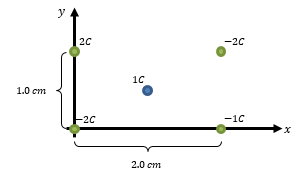# Problem: What is the net electric force on the 1 C charge? Consider the 1 C to placed directly in the center of the arrangement.

###### Problem Details

What is the net electric force on the 1 C charge? Consider the 1 C to placed directly in the center of the arrangement.What scientific concept do you need to know in order to solve this problem?

Our tutors have indicated that to solve this problem you will need to apply the Coulomb's Law (Electric Force) concept. You can view video lessons to learn Coulomb's Law (Electric Force). Or if you need more Coulomb's Law (Electric Force) practice, you can also practice Coulomb's Law (Electric Force) practice problems.

What is the difficulty of this problem?

Our tutors rated the difficulty ofWhat is the net electric force on the 1 C charge? Consider t...as medium difficulty.

How long does this problem take to solve?

Our expert Physics tutor, Juan took 7 minutes and 23 seconds to solve this problem. You can follow their steps in the video explanation above.

What professor is this problem relevant for?

Based on our data, we think this problem is relevant for Professor Dukes' class at UVA.